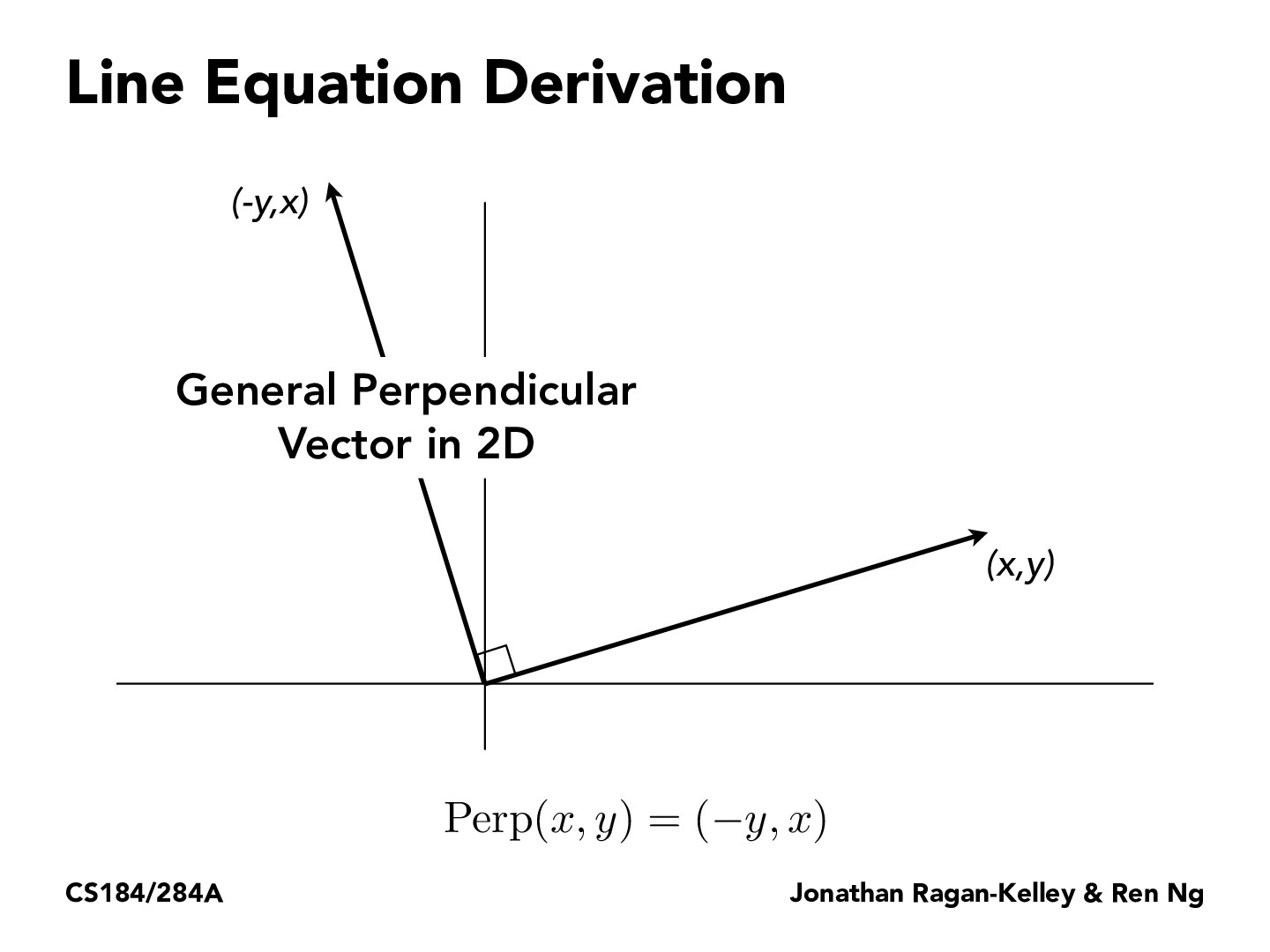Lecture 2: Rasterization (38)ponedo

The normal vector generated by this mean points "left" side of the original vector. In this case, we should take vertexes in counterclockwise order to get the vectors corresponding to every edge so that we can perform the Inside-Triangle test in the next pages correctly.

If we modify the equation as Perp(x, y) = (y, -x) just adopt clockwise order and the Inside-Triangle test would still work.jchen12197

I understand that we can use the line equation on all three sides of a triangle to test whether a point is inside of the triangle, but I don't understand the derivation. How does using this perpendicular vector allow us to decide if a point is on a certain side of the line? And how do we know which side of the line is "inside" (i.e. if the point falls in the shaded orange area on the next few slides)?jeshlee121

You are right that we don't know which side of the line is "inside" (we don't know which face of the triangle we are looking at); however we know that if for all the sides, the point is inside the line, this means the point is inside the triangle. Similarly, if the point is outside all three sides, this is physically impossible, and must mean that we are looking at the other face of the triangle, and in this case the point is also inside the triangle.

You must be enrolled in the course to comment